# Normal Distributions 22712 Normal Distribution Central Limit Theorem

• Slides: 38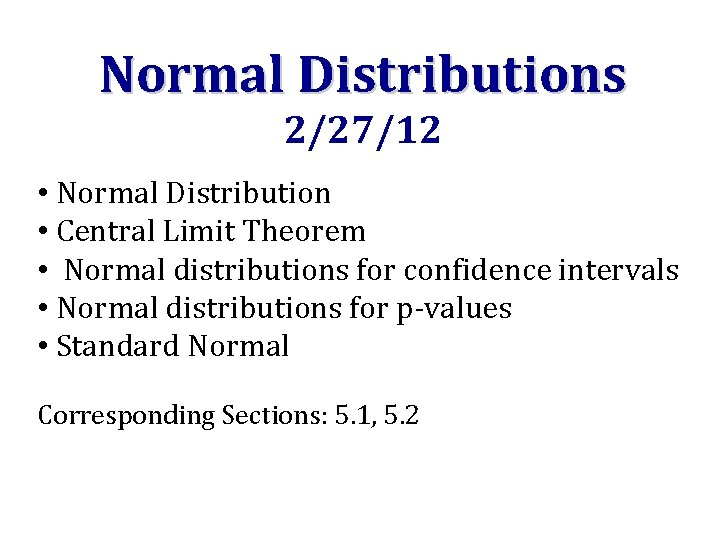Normal Distributions 2/27/12 • Normal Distribution • Central Limit Theorem • Normal distributions for confidence intervals • Normal distributions for p-values • Standard Normal Corresponding Sections: 5. 1, 5. 2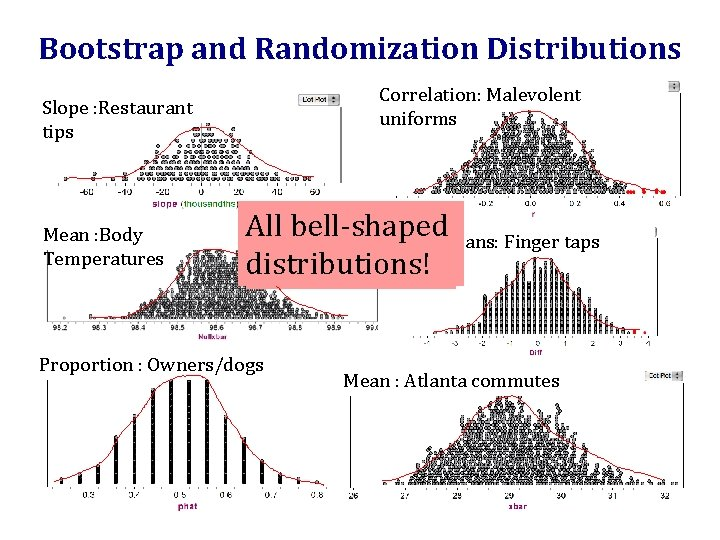Bootstrap and Randomization Distributions Correlation: Malevolent uniforms Slope : Restaurant tips Mean : Body Temperatures All bell-shaped What do you Diff means: Finger taps distributions! notice? Proportion : Owners/dogs Mean : Atlanta commutesNormal Distribution • The symmetric, bell-shaped curve we have seen for almost all of our bootstrap and randomization distributions is called a normal distributionCentral Limit Theorem! For a sufficiently large sample size, the distribution of sample statistics for a mean or a proportion is normal http: //onlinestatbook. com/stat_sim/sampling_dist/index. html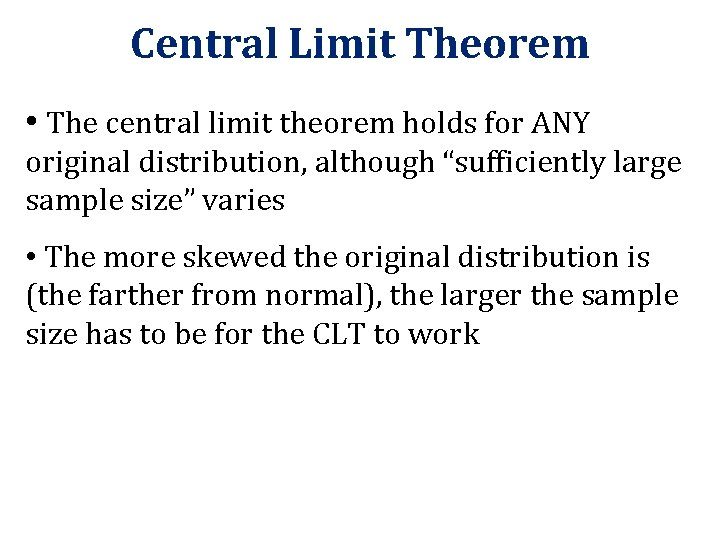Central Limit Theorem • The central limit theorem holds for ANY original distribution, although “sufficiently large sample size” varies • The more skewed the original distribution is (the farther from normal), the larger the sample size has to be for the CLT to work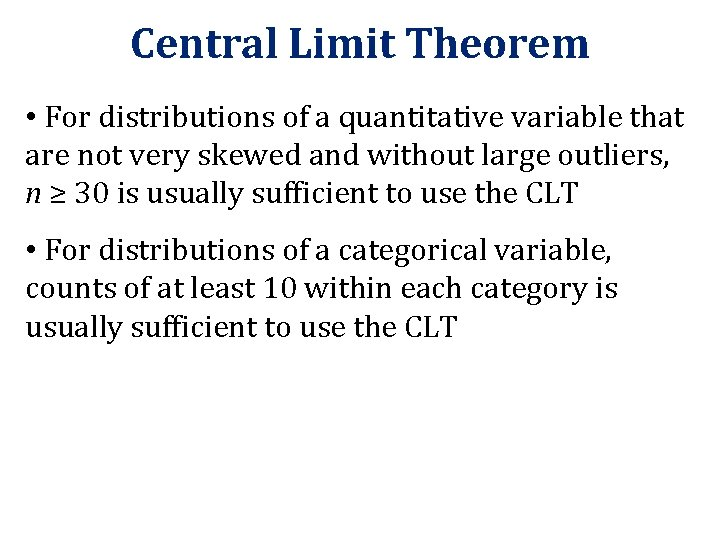Central Limit Theorem • For distributions of a quantitative variable that are not very skewed and without large outliers, n ≥ 30 is usually sufficient to use the CLT • For distributions of a categorical variable, counts of at least 10 within each category is usually sufficient to use the CLTNormal Distribution • The normal distribution is fully characterized by it’s mean and standard deviation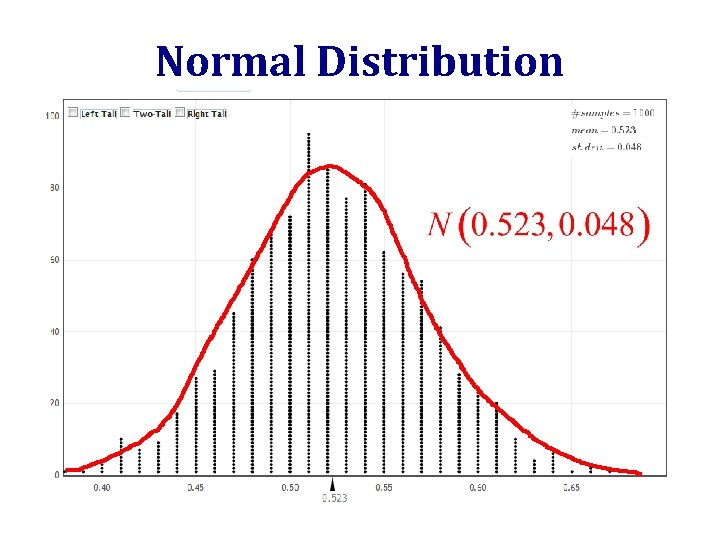Normal Distribution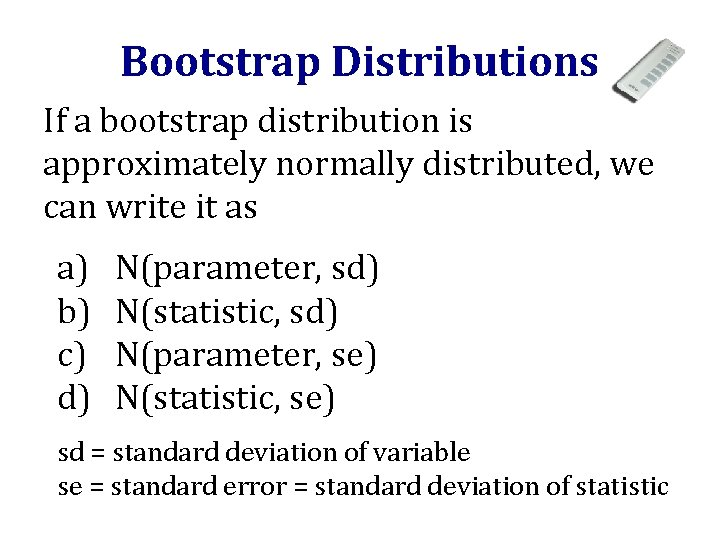Bootstrap Distributions If a bootstrap distribution is approximately normally distributed, we can write it as a) b) c) d) N(parameter, sd) N(statistic, sd) N(parameter, se) N(statistic, se) sd = standard deviation of variable se = standard error = standard deviation of statistic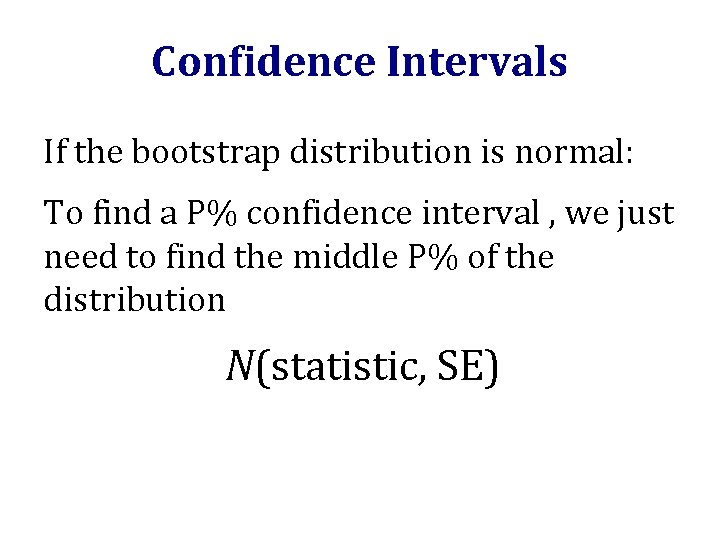Confidence Intervals If the bootstrap distribution is normal: To find a P% confidence interval , we just need to find the middle P% of the distribution N(statistic, SE)Best Picture What proportion of visitors to www. naplesnews. com thought The Artist should win best picture?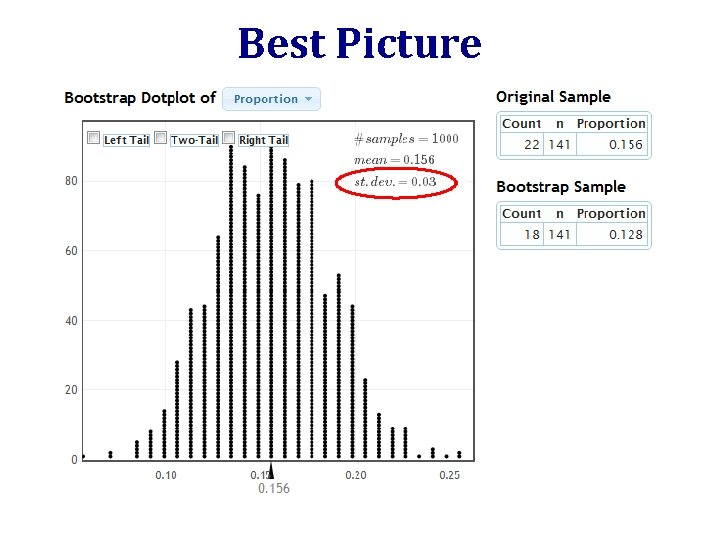Best Picture www. lock 5 stat. com/statkeyArea under a Curve • The area under the curve of a normal distribution is equal to the proportion of the distribution falling within that range • Knowing just the mean and standard deviation of a normal distribution allows you to calculate areas in the tails and percentiles http: //davidmlane. com/hyperstat/z_table. html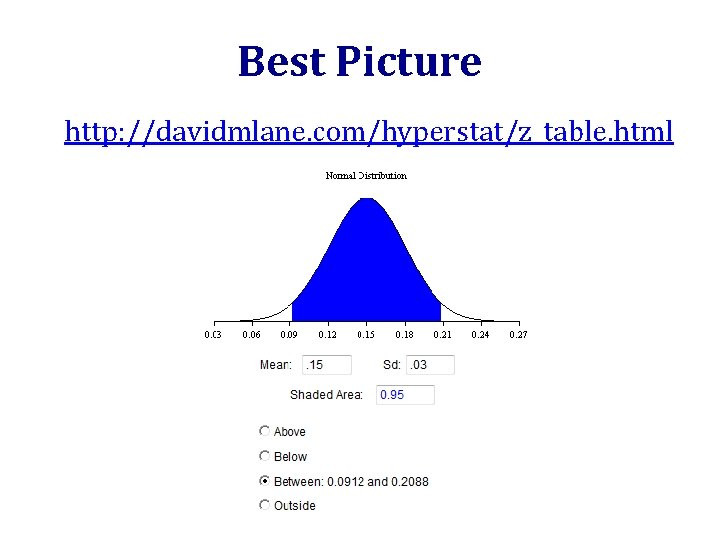Best Picture http: //davidmlane. com/hyperstat/z_table. htmlBest PictureConfidence Intervals For a normal sampling distribution, we can also use the formula to give a 95% confidence interval.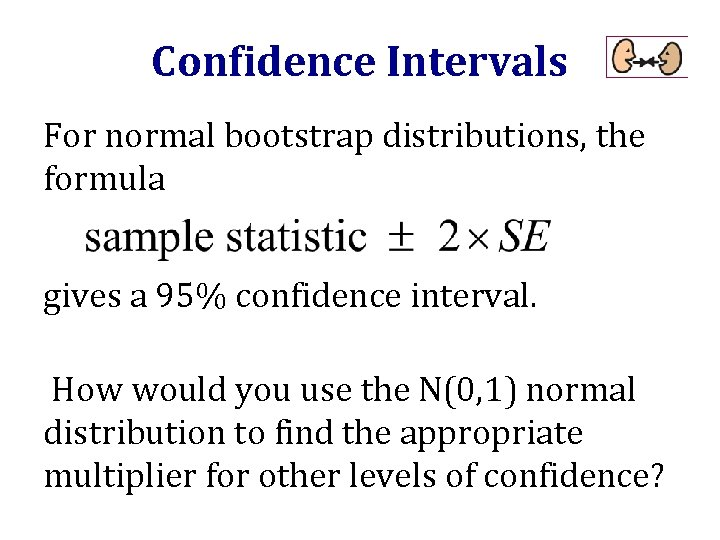Confidence Intervals For normal bootstrap distributions, the formula gives a 95% confidence interval. How would you use the N(0, 1) normal distribution to find the appropriate multiplier for other levels of confidence?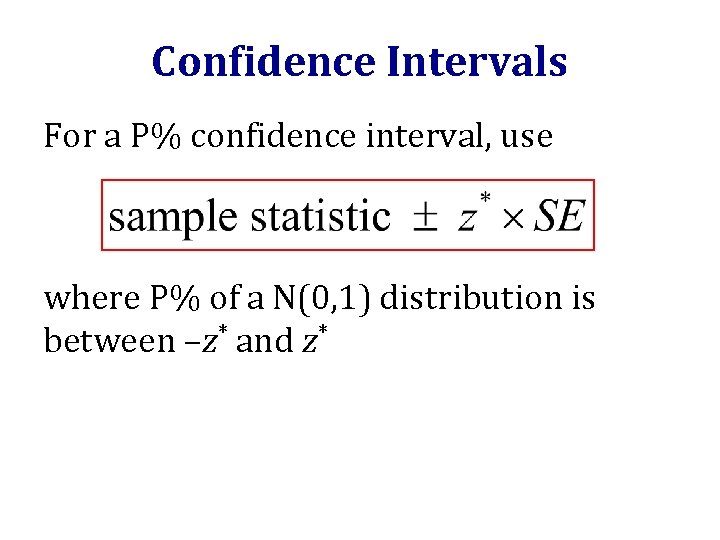Confidence Intervals For a P% confidence interval, use where P% of a N(0, 1) distribution is between –z* and z*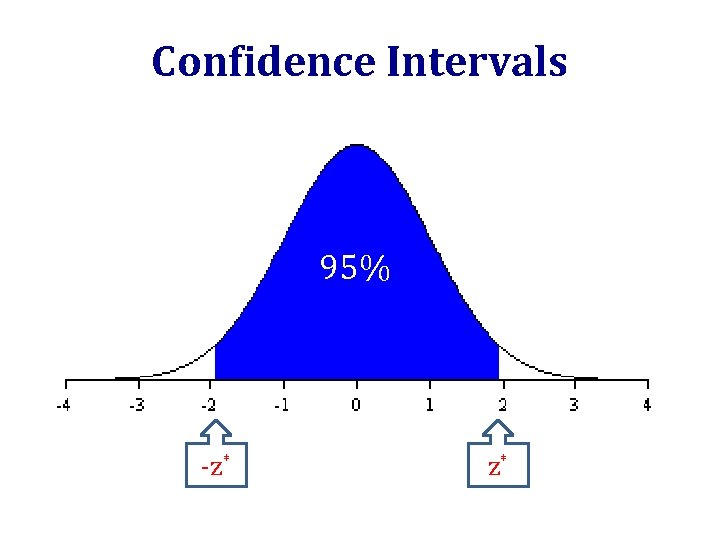Confidence Intervals 95% -z* z*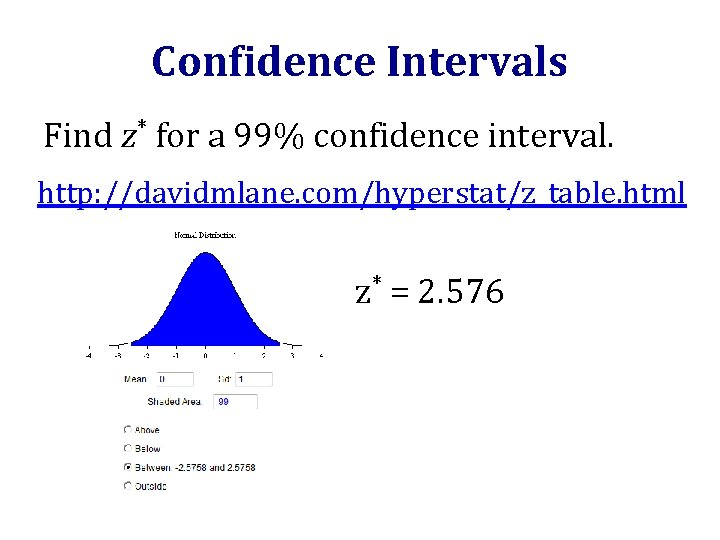Confidence Intervals Find z* for a 99% confidence interval. http: //davidmlane. com/hyperstat/z_table. html z* = 2. 576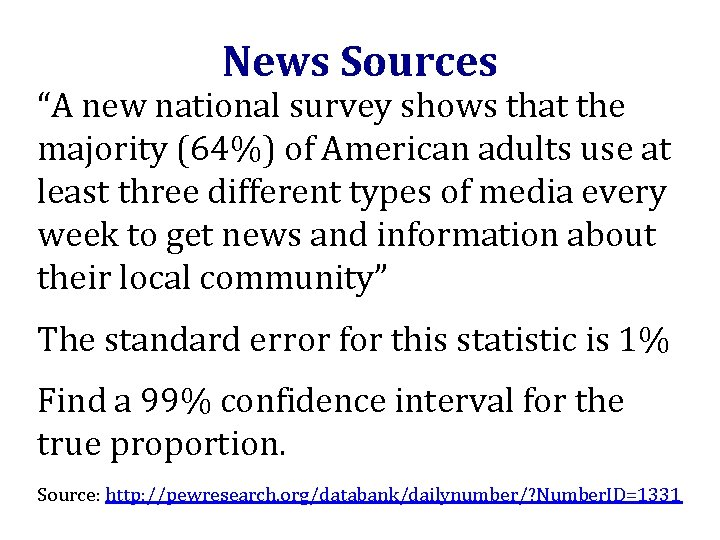News Sources “A new national survey shows that the majority (64%) of American adults use at least three different types of media every week to get news and information about their local community” The standard error for this statistic is 1% Find a 99% confidence interval for the true proportion. Source: http: //pewresearch. org/databank/dailynumber/? Number. ID=1331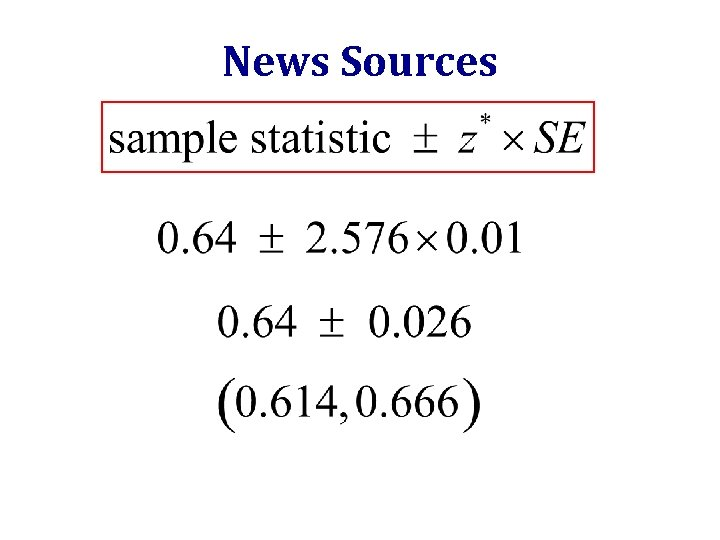News Sources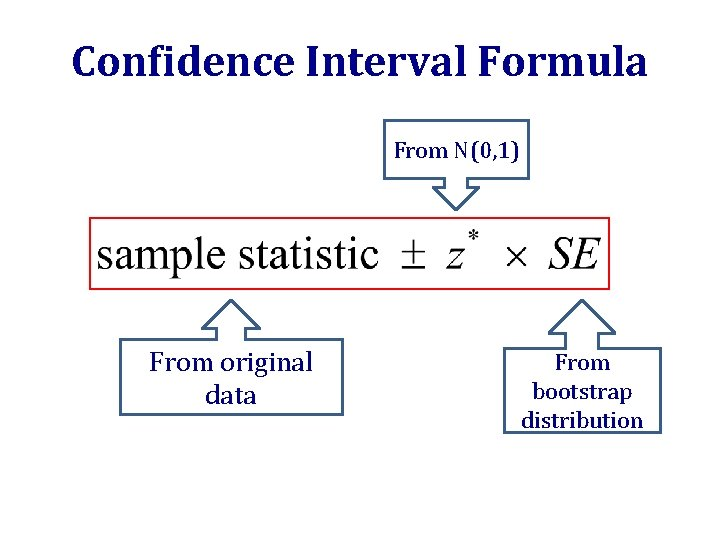Confidence Interval Formula From N(0, 1) From original data From bootstrap distribution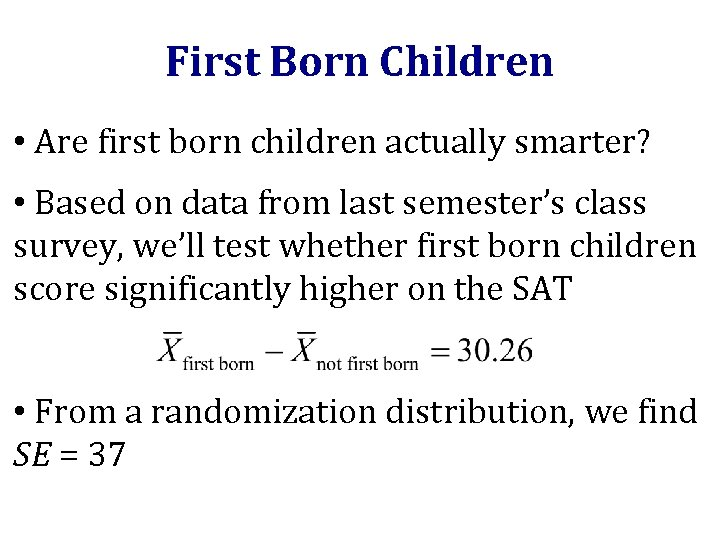First Born Children • Are first born children actually smarter? • Based on data from last semester’s class survey, we’ll test whether first born children score significantly higher on the SAT • From a randomization distribution, we find SE = 37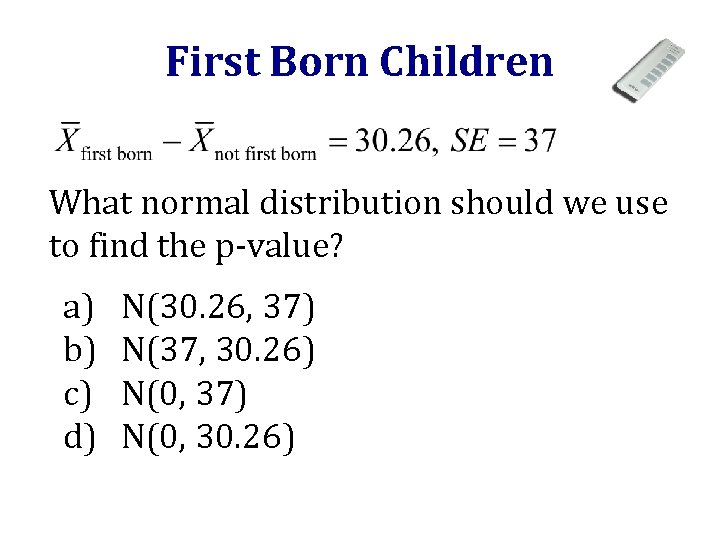First Born Children What normal distribution should we use to find the p-value? a) b) c) d) N(30. 26, 37) N(37, 30. 26) N(0, 37) N(0, 30. 26)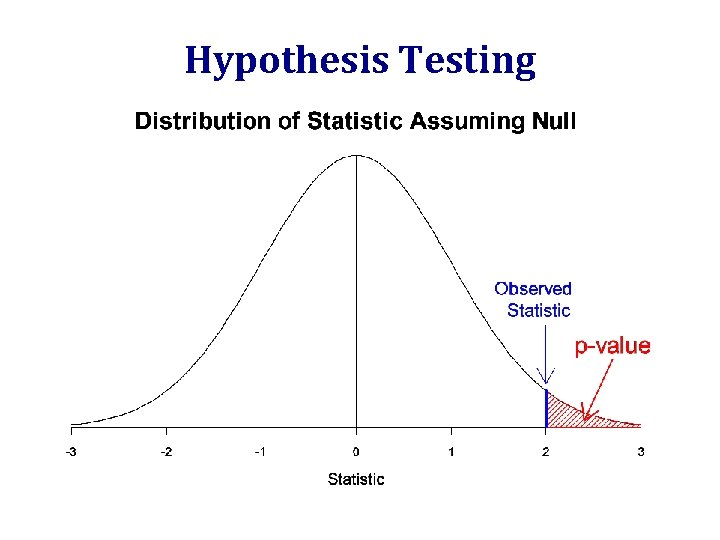Hypothesis Testingp-values If the randomization distribution is normal: To calculate a p-value, we just need to find the area in the appropriate tail(s) beyond the observed statistic of the distribution N(null value, SE)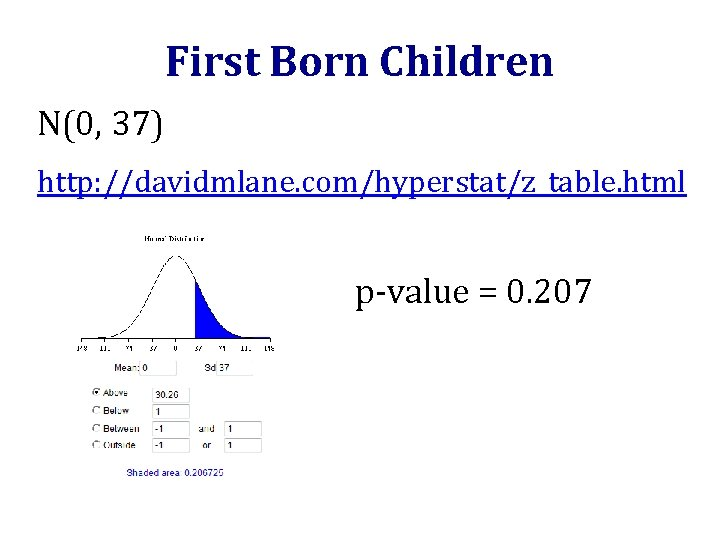First Born Children N(0, 37) http: //davidmlane. com/hyperstat/z_table. html p-value = 0. 207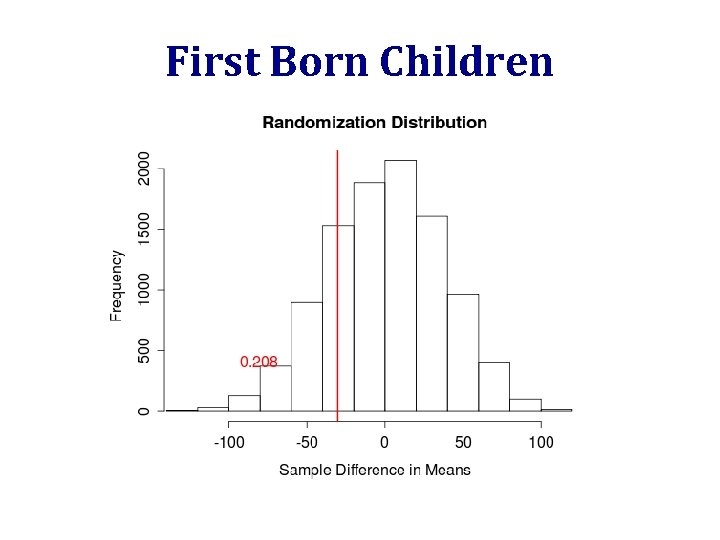First Born Children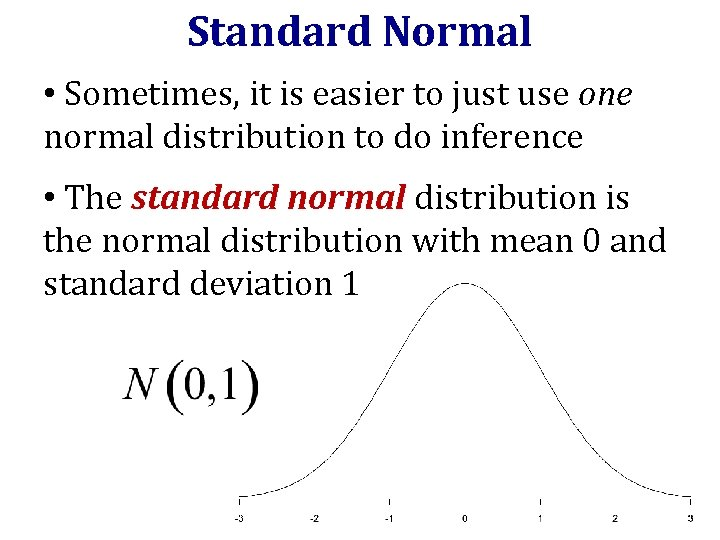Standard Normal • Sometimes, it is easier to just use one normal distribution to do inference • The standard normal distribution is the normal distribution with mean 0 and standard deviation 1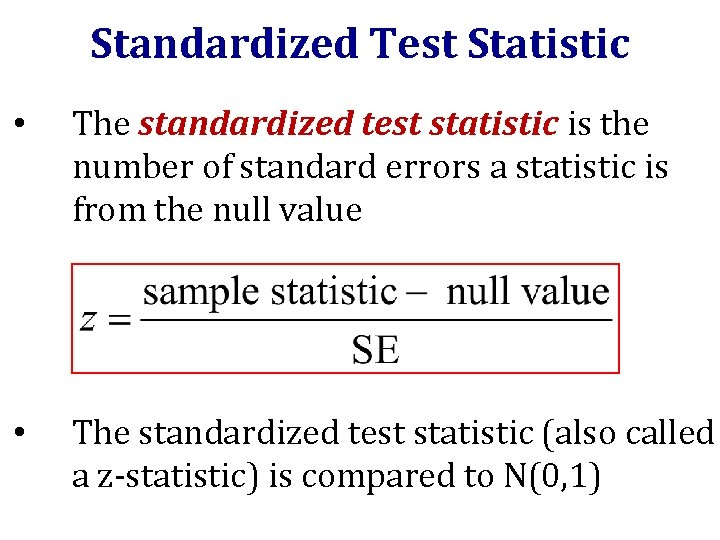Standardized Test Statistic • The standardized test statistic is the number of standard errors a statistic is from the null value • The standardized test statistic (also called a z-statistic) is compared to N(0, 1)p-value 1) Find the standardized test statistic: 2) The p-value is the area in the tail(s) beyond z for a standard normal distributionFirst Born Children 1) Find the standardized test statisticFirst Born Children 2) Find the area in the tail(s) beyond z for a standard normal distribution p-value = 0. 207z-statistic • Calculating the number of standard errors a statistic is from the null value allows us to assess extremity on a common scale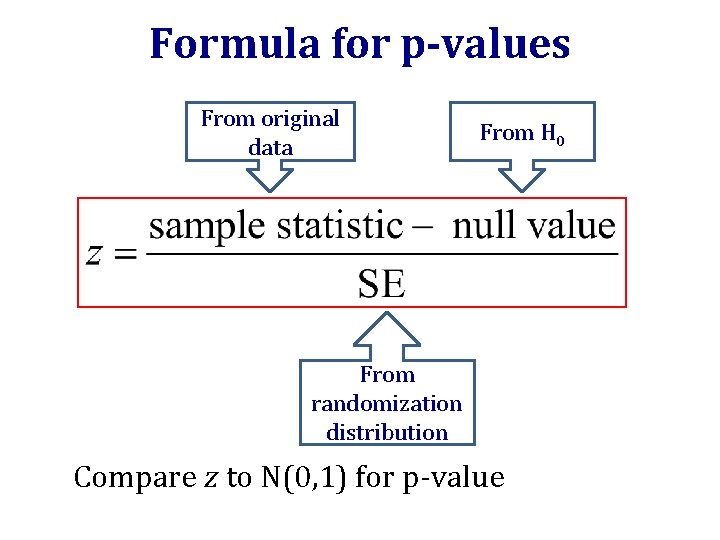Formula for p-values From original data From H 0 From randomization distribution Compare z to N(0, 1) for p-valueStandard Error • Wouldn’t it be nice if we could compute the standard error without doing thousands of simulations? • We can!!! • Or rather, we’ll be able to on Wednesday!